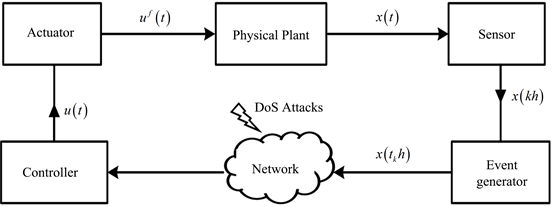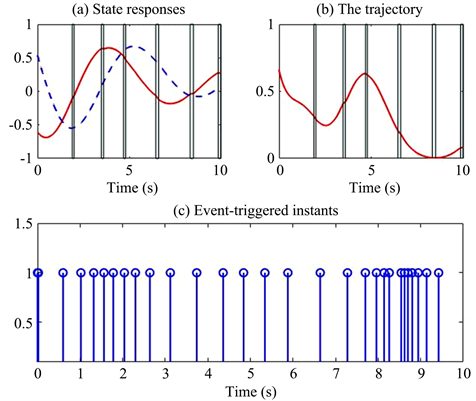#### 期刊菜单

DoS攻击下不确定网络控制系统基于事件触发机制的鲁棒有限时间可靠控制
Robust Finite-Time Reliable Control for Uncertain Networked Control Systems under Denial of Service Attacks Based on Event Triggering Mechanism
DOI: 10.12677/DSC.2022.113012, PDF, HTML, XML, 下载: 134  浏览: 641  国家自然科学基金支持

Abstract: In this paper, robust finite-time stability of Networked Control Systems (NCSs) based on event triggering mechanism under actuator failures and non-periodic Denial of Service (DoS) attacks is investigated. First, a switching-like delay system model is presented under discrete event-triggered communication scheme. Then, by constructing piecewise Lyapunov-Krasovskii functional, sufficient conditions for finite-time stability of the system are derived and expressed with a set of linear matrix inequalities. Moreover, the co-design of the robust reliable controller and the event-triggered parameter is obtained. Finally, two examples are employed to demonstrate the effectiveness of the proposed method.

1. 引言

2. 问题描述

$\stackrel{˙}{x}\left(t\right)=\left(A+\Delta A\left(t\right)\right)x\left(t\right)+\left(B+\Delta B\left(t\right)\right){u}^{f}\left(t\right),$ (1)

$\Delta A\left(t\right)=EH\left(t\right){F}_{1},\Delta B\left(t\right)=EH\left(t\right){F}_{2},$ (2)

${u}^{f}\left(t\right)=\Xi u\left(t\right),$ (3)

$\Xi =diag\left\{{d}_{1},{d}_{2},\cdots ,{d}_{m}\right\},$

$U=diag\left\{{u}_{1},{u}_{2},\cdots ,{u}_{m}\right\},L=diag\left\{{p}_{1},{p}_{2},\cdots ,{p}_{m}\right\},E=diag\left\{{e}_{1},{e}_{2},\cdots ,{e}_{m}\right\}$

$\Xi =U\left(I+E\right),|E|\le L\le I,$ (4)

${I}_{DOS}\left(t\right)=\left\{\begin{array}{l}0,\text{\hspace{0.17em}}\text{\hspace{0.17em}}t\in \left[{h}_{n},{h}_{n}+{l}_{n}\right)\\ 1,\text{\hspace{0.17em}}\text{\hspace{0.17em}}\text{ }t\in \left[{h}_{n}+{l}_{n},{h}_{n+1}\right).\end{array}$ (5)Figure 1. Structure diagram of NCSs based on event triggering mechanism

$u\left(t\right)=Kx\left({t}_{k}h\right),t\in \left[{t}_{k}h,{t}_{k+1}h\right),$ (6)

${e}_{k,j}^{\text{T}}\left({t}_{{k}_{j}}h\right)\Phi {e}_{k,j}\left({t}_{{k}_{j}}h\right)\ge \delta {x}^{\text{T}}\left({t}_{k}h\right)\Phi x\left({t}_{k}h\right),$ (7)

${t}_{k,n}h=\left\{{t}_{{k}_{j}}h\text{ }\text{ }满足\text{(7)}|{t}_{{k}_{j}}h\in {L}_{1,n-1}\right\}\cup \left\{{h}_{n}\right\},$ (8)

$u\left(t\right)=\left\{\begin{array}{l}Kx\left({t}_{k,n}h\right),\text{\hspace{0.17em}}\text{\hspace{0.17em}}t\in \left[{t}_{k,n}h,{t}_{k+1,n}h\right)\cap {L}_{1,n-1}\\ 0,\text{\hspace{0.17em}}\text{\hspace{0.17em}}t\in {L}_{2,n-1},\end{array}$ (9)

$\stackrel{˙}{x}\left(t\right)=\left\{\begin{array}{l}\left(A+\Delta A\left(t\right)\right)x\left(t\right)+\left(B+\Delta B\left(t\right)\right)\Xi Kx\left({t}_{k,n}h\right),\text{\hspace{0.17em}}\text{\hspace{0.17em}}t\in {\nu }_{k,n}\cap {L}_{1,n-1}\\ \left(A+\Delta A\left(t\right)\right)x\left(t\right),\text{\hspace{0.17em}}\text{\hspace{0.17em}}t\in {L}_{2,n-1}.\end{array}$ (10)

${\nu }_{k,n}={\cup }_{l=1}^{{\lambda }_{k,n}+1}{F}_{k,n}^{l},$ (11)

${F}_{k,n}^{l}=\left[{t}_{k,n}h+\left(l-1\right)h,{t}_{k,n}h+lh\right),l=1,2,\cdots ,{\lambda }_{k,n},$

${\lambda }_{k,n}\triangleq \mathrm{sup}\left\{l\in ℕ|{t}_{k,n}h+lh<{t}_{k+1,n}h\right\},$

${F}_{k,n}^{{\lambda }_{k,n}+1}=\left[{t}_{k,n}h+{\lambda }_{k,n}h,{t}_{k+1,n}h\right).$

${L}_{1,n-1}\subseteq {\cup }_{k=0}^{k\left(n\right)}{\nu }_{k,n},$ (12)

${L}_{1,n-1}={\cup }_{k=0}^{k\left(n\right)}\left\{{\upsilon }_{k,n}\cap {L}_{1,n-1}\right\}={\cup }_{k=0}^{k\left(n\right)}{\cup }_{l=1}^{{\lambda }_{k,n}+1}\left\{{F}_{k,n}^{l}\cap {L}_{1,n-1}\right\}.$

${\varphi }_{k,n}^{l}={F}_{k,n}^{l}\cap {L}_{1,n-1}$，则 ${L}_{1,n-1}={\cup }_{k=0}^{k\left(n\right)}{\cup }_{l=1}^{{\lambda }_{k,n}+1}{\varphi }_{k,n}^{l}$

${\tau }_{k,n}\left(t\right)=\left\{\begin{array}{l}t-{t}_{k,n}h,\text{\hspace{0.17em}}\text{\hspace{0.17em}}t\in {\varphi }_{k,n}^{1}\\ t-{t}_{k,n}h-h,\text{\hspace{0.17em}}\text{\hspace{0.17em}}t\in {\varphi }_{k,n}^{2}\\ \text{\hspace{0.17em}}\text{\hspace{0.17em}}\text{\hspace{0.17em}}\text{\hspace{0.17em}}\text{\hspace{0.17em}}\text{\hspace{0.17em}}\text{\hspace{0.17em}}\text{\hspace{0.17em}}\text{\hspace{0.17em}}\text{\hspace{0.17em}}\text{\hspace{0.17em}}⋮\\ t-{t}_{k,n}h-{\lambda }_{k,n}h,\text{\hspace{0.17em}}\text{\hspace{0.17em}}t\in {\varphi }_{k,n}^{{\lambda }_{k,n}+1},\end{array}$

${e}_{k,n}\left(t\right)=\left\{\begin{array}{l}0,\text{\hspace{0.17em}}\text{\hspace{0.17em}}t\in {\varphi }_{k,n}^{1}\\ x\left({t}_{k,n}h\right)-x\left({t}_{k,n}h+h\right),\text{\hspace{0.17em}}\text{\hspace{0.17em}}t\in {\varphi }_{k,n}^{2}\\ \text{\hspace{0.17em}}\text{\hspace{0.17em}}\text{\hspace{0.17em}}\text{\hspace{0.17em}}\text{\hspace{0.17em}}\text{\hspace{0.17em}}\text{\hspace{0.17em}}\text{\hspace{0.17em}}\text{\hspace{0.17em}}\text{\hspace{0.17em}}\text{\hspace{0.17em}}\text{\hspace{0.17em}}⋮\\ x\left({t}_{k,n}h\right)-x\left({t}_{k,n}h+{\lambda }_{k,n}h\right),\text{\hspace{0.17em}}\text{\hspace{0.17em}}t\in {\varphi }_{k,n}^{{\lambda }_{k,n}+1},\end{array}$

$x\left({t}_{k,n}h\right)=x\left(t-{\tau }_{k,n}\left(t\right)\right)+{e}_{k,n}\left(t\right),t\in {\nu }_{n,k}\cap {L}_{1,n-1}.$ (13)

$\left\{\begin{array}{l}\stackrel{˙}{x}\left(t\right)=\left\{\begin{array}{l}\left(A+\Delta A\left(t\right)\right)x\left(t\right)+\left(B+\Delta B\left(t\right)\right)\Xi Kx\left(t-{\tau }_{k,n}\left(t\right)\right)+\left(B+\Delta B\left(t\right)\right)\Xi K{e}_{k,n}\left(t\right),\text{\hspace{0.17em}}\text{\hspace{0.17em}}t\in {\nu }_{k,n}\cap {L}_{1,n-1}\\ \left(A+\Delta A\left(t\right)\right)x\left(t\right),\text{\hspace{0.17em}}\text{\hspace{0.17em}}t\in {L}_{2,n-1}\end{array}\\ x\left(t\right)=\varphi \left(t\right),\text{\hspace{0.17em}}\text{\hspace{0.17em}}t\in \left[-h,0\right],\end{array}$ (14)

$\underset{\theta \in \left[-h,0\right]}{\mathrm{sup}}\left\{{x}^{\text{T}}\left(\theta \right)Rx\left(\theta \right),{\stackrel{˙}{x}}^{\text{T}}\left(\theta \right)R\stackrel{˙}{x}\left(\theta \right)\right\}\le {c}_{1}⇒{x}^{\text{T}}\left(t\right)Rx\left(t\right)<{c}_{2}\text{,}\forall t\in \left[0,{T}_{f}\right],$

$-{\tau }_{M}{\int }_{t-{\tau }_{M}}^{t}{\stackrel{˙}{x}}^{\text{T}}\left(s\right)Q\stackrel{˙}{x}\left(s\right)\text{d}s\le {\left[\begin{array}{c}{x}^{\text{T}}\left(t\right)\\ {x}^{\text{T}}\left(t-\tau \left(t\right)\right)\\ {x}^{\text{T}}\left(t-{\tau }_{M}\right)\end{array}\right]}^{\text{T}}\left[\begin{array}{ccc}-Q& Q-U& -U\\ *& -2Q+U+{U}^{\text{T}}& Q-U\\ *& *& -Q\end{array}\right]\left[\begin{array}{c}x\left(t\right)\\ x\left(t-\tau \left(t\right)\right)\\ x\left(t-{\tau }_{M}\right)\end{array}\right].$

$X+UVW+{W}^{\text{T}}{V}^{\text{T}}{U}^{\text{T}}<0,$

$X+\rho U{U}^{\text{T}}+{\rho }^{-1}{W}^{\text{T}}W<0.$

3. 主要结果

$\left\{\begin{array}{l}\stackrel{˙}{x}\left(t\right)=\left\{\begin{array}{l}Ax\left(t\right)+B\Xi Kx\left(t-{\tau }_{k,n}\left(t\right)\right)+B\Xi K{e}_{k,n}\left(t\right),\text{\hspace{0.17em}}\text{\hspace{0.17em}}t\in {\nu }_{k,n}\cap {L}_{1,n-1}\\ Ax\left(t\right),\text{\hspace{0.17em}}\text{\hspace{0.17em}}t\in {L}_{2,n-1}\end{array}\\ x\left(t\right)=\phi \left(t\right),\text{\hspace{0.17em}}\text{\hspace{0.17em}}t\in \left[-h,0\right].\end{array}$ (15)

3.1. 有限时间稳定性分析

$V\left(t\right)=\left\{\begin{array}{l}{V}_{1}\left(t\right),\text{\hspace{0.17em}}\text{\hspace{0.17em}}t\in {\nu }_{k,n}\cap {L}_{1,n-1}\\ {V}_{2}\left(t\right),\text{\hspace{0.17em}}\text{\hspace{0.17em}}t\in {L}_{2,n-1},\end{array}$

${\Pi }_{1}<0,\text{\hspace{0.17em}}\text{\hspace{0.17em}}{\Sigma }_{1}>0,$ (16)

${\Pi }_{2}<0,\text{\hspace{0.17em}}\text{\hspace{0.17em}}{\Sigma }_{2}>0,$ (17)

${\Sigma }_{1}=\left[\begin{array}{cc}{Z}_{1}& {S}_{1}\\ *& {Z}_{1}\end{array}\right],\text{}{\Sigma }_{2}=\left[\begin{array}{cc}{Z}_{2}& {S}_{2}\\ *& {Z}_{2}\end{array}\right],\text{}{\Pi }_{1}=\left[\begin{array}{ccc}\stackrel{˜}{\Upsilon }& \phi & \psi \\ *& -{\rho }^{-1}I& 0\\ *& 0& -\rho I\end{array}\right],\text{}\stackrel{˜}{\Upsilon }=\left[\begin{array}{cc}\stackrel{˜}{\Theta }& h{\stackrel{˜}{\Gamma }}_{1}^{\text{T}}{Z}_{1}\\ *& -{Z}_{1}\end{array}\right],\text{}{\stackrel{˜}{\Gamma }}_{1}^{\text{T}}=\left[\begin{array}{c}{A}^{\text{T}}\\ {\left(BUK\right)}^{\text{T}}\\ 0\\ {\left(BUK\right)}^{\text{T}}\end{array}\right],$

$\phi =\left[\begin{array}{c}{P}_{1}B\\ 0\\ 0\\ \begin{array}{l}\text{}0\\ h{Z}_{1}B\end{array}\end{array}\right],\text{}\psi =\left[\begin{array}{c}0\\ {K}^{\text{T}}{U}^{\text{T}}\\ 0\\ \begin{array}{l}{K}^{\text{T}}{U}^{\text{T}}\\ \text{}0\end{array}\end{array}\right],\text{}\stackrel{˜}{\Theta }=\left[\begin{array}{cccc}{\stackrel{˜}{\Theta }}_{11}& {\stackrel{˜}{\Theta }}_{12}& {\stackrel{˜}{\Theta }}_{13}& {\stackrel{˜}{\Theta }}_{14}\\ *& {\stackrel{˜}{\Theta }}_{22}& {\stackrel{˜}{\Theta }}_{23}& {\stackrel{˜}{\Theta }}_{24}\\ *& *& {\stackrel{˜}{\Theta }}_{33}& 0\\ *& *& *& \text{}{\stackrel{˜}{\Theta }}_{44}\end{array}\right],\text{}{\Pi }_{2}=\left[\begin{array}{cccc}{\Omega }_{11}& {\Omega }_{12}& {\Omega }_{13}& h{A}^{\text{T}}{Z}_{2}\\ *& {\Omega }_{22}& {\Omega }_{23}& 0\\ *& *& {\Omega }_{33}& 0\\ *& *& *& -{Z}_{2}\end{array}\right],$

$\begin{array}{l}{\stackrel{˜}{\Theta }}_{11}={A}^{\text{T}}{P}_{1}+{P}_{1}A+{Q}_{1}+2{\alpha }_{1}{P}_{1}-{\text{e}}^{-{\alpha }_{1}h}{Z}_{1},\text{}{\stackrel{˜}{\Theta }}_{12}={P}_{1}BUK-{\text{e}}^{-{\alpha }_{1}h}\left({S}_{1}-{Z}_{1}\right),\text{}{\stackrel{˜}{\Theta }}_{13}={\text{e}}^{-{\alpha }_{1}h}{S}_{1},\text{}{\stackrel{˜}{\Theta }}_{14}={P}_{1}BUK,\\ {\stackrel{˜}{\Theta }}_{22}=\delta \text{ }\Phi -{\text{e}}^{-{\alpha }_{1}h}\left(2{Z}_{1}-{S}_{1}-{S}_{1}^{\text{T}}\right),\text{}{\stackrel{˜}{\Theta }}_{23}=-{\text{e}}^{-{\alpha }_{1}h}\left({S}_{1}-{Z}_{1}\right),\text{}{\stackrel{˜}{\Theta }}_{24}=\delta \text{ }\Phi ,\text{}{\stackrel{˜}{\Theta }}_{33}=-{\text{e}}^{-{\alpha }_{1}h}\left({Q}_{1}+{Z}_{1}\right),\text{}{\stackrel{˜}{\Theta }}_{44}=\delta \text{ }\Phi -\Phi ,\\ {\Omega }_{11}={A}^{\text{T}}{P}_{2}+{P}_{2}A+{Q}_{2}-{\alpha }_{2}{P}_{2}-{Z}_{2},\text{}{\Omega }_{12}={Z}_{2}-{S}_{2},\text{}{\Omega }_{13}={S}_{2},\text{}{\Omega }_{22}={S}_{2}+{S}_{2}^{\text{T}}-2{Z}_{2},\text{}{\Omega }_{23}={Z}_{2}-{S}_{2},\\ {\Omega }_{33}=-{\text{e}}^{{\alpha }_{2}h}{Q}_{2}-{Z}_{2},\end{array}$

$V\left(t\right)\le \left\{\begin{array}{l}{\text{e}}^{-{\alpha }_{1}\left(t-{h}_{n-1}\right)}{V}_{1}\left({h}_{n-1}\right),\text{\hspace{0.17em}}\text{\hspace{0.17em}}t\in {\nu }_{k,n}\cap {L}_{1,n-1}\\ {\text{e}}^{{\alpha }_{2}\left(t-{h}_{n-1}-{l}_{n-1}\right)}{V}_{2}\left({h}_{n-1}+{l}_{n-1}\right),\text{\hspace{0.17em}}\text{\hspace{0.17em}}t\in {L}_{2,n-1}.\end{array}$ (18)

(i) $\forall t\in {\nu }_{k,n}\cap {L}_{1,n-1}$，误差 ${e}_{k,n}\left(t\right)$ 满足

${e}_{k,n}^{\text{T}}\left(t\right)\Phi {e}_{k,n}\left(t\right)\le \delta {\left[x\left(t-{\tau }_{k,n}\left(t\right)\right)+{e}_{k,n}\left(t\right)\right]}^{\text{T}}\Phi \left[x\left(t-{\tau }_{k,n}\left(t\right)\right)+{e}_{k,n}\left(t\right)\right].$ (19)

${V}_{1}\left(t\right)$ 沿着系统(17)的轨迹关于t求导可得

$\begin{array}{c}{\stackrel{˙}{V}}_{1}\left(t\right)=-{\alpha }_{1}V\left(t\right)+{\alpha }_{1}{x}^{\text{T}}\left(t\right){P}_{1}x\left(t\right)+2{x}^{\text{T}}\left(t\right){P}_{1}\stackrel{˙}{x}\left(t\right)-{x}^{\text{T}}\left(t-h\right){\text{e}}^{-{\alpha }_{1}h}{P}_{1}x\left(t-h\right)\\ \text{\hspace{0.17em}}\text{ }\text{ }+{h}^{2}{\stackrel{˙}{x}}^{\text{T}}\left(t\right){Z}_{1}\stackrel{˙}{x}\left(t\right)-h{\int }_{t-h}^{t}{\stackrel{˙}{x}}^{\text{T}}\left(s\right){\text{e}}^{-{\alpha }_{1}\left(t-s\right)}{Z}_{1}\stackrel{˙}{x}\left(s\right)\text{d}s\\ \le -{\alpha }_{1}V\left(t\right)+{\alpha }_{1}{x}^{\text{T}}\left(t\right){P}_{1}x\left(t\right)+2{x}^{\text{T}}\left(t\right){P}_{1}\stackrel{˙}{x}\left(t\right)-{x}^{\text{T}}\left(t-h\right){\text{e}}^{-{\alpha }_{1}h}{P}_{1}x\left(t-h\right)\\ \text{\hspace{0.17em}}\text{ }\text{ }+{h}^{2}{\stackrel{˙}{x}}^{\text{T}}\left(t\right){Z}_{1}\stackrel{˙}{x}\left(t\right)-h{\text{e}}^{-{\alpha }_{1}h}{\int }_{t-h}^{t}{\stackrel{˙}{x}}^{\text{T}}\left(s\right){Z}_{1}\stackrel{˙}{x}\left(s\right)\text{d}s.\end{array}$ (20)

$-h{\int }_{t-h}^{t}{\stackrel{˙}{x}}^{\text{T}}\left(s\right){Z}_{1}\stackrel{˙}{x}\left(s\right)\text{d}s\le -{\varsigma }^{\text{T}}\left(t\right)\left[\begin{array}{ccc}{Z}_{1}& {S}_{1}-{Z}_{1}& -{S}_{1}\\ *& 2{Z}_{1}-{S}_{1}-{S}_{1}^{\text{T}}& {S}_{1}-{Z}_{1}\\ *& *& {Z}_{1}\end{array}\right]\varsigma \left(t\right).$ (21)

${\stackrel{˙}{V}}_{1}\left(t\right)\le -{\alpha }_{1}V\left(t\right)+{\xi }^{\text{T}}\left(t\right)\left(\Theta +{h}^{2}{\Gamma }_{1}^{\text{T}}{Z}_{1}{\Gamma }_{1}\right)\xi \left(t\right),$ (22)

$\xi \left(t\right)={\left[{x}^{\text{T}}\left(t\right),{x}^{\text{T}}\left(t-{\tau }_{k,n}\left(t\right)\right),{x}^{\text{T}}\left(t-h\right),{e}_{k,n}^{\text{T}}\left(t\right)\right]}^{\text{T}},$

$\Theta =\left[\begin{array}{cccc}{\Theta }_{11}& {\Theta }_{12}& {\Theta }_{13}& {\Theta }_{14}\\ *& {\Theta }_{22}& {\Theta }_{23}& {\Theta }_{24}\\ *& *& {\Theta }_{33}& 0\\ *& *& *& {\Theta }_{44}\end{array}\right],\text{\hspace{0.17em}}{\Gamma }_{1}^{\text{T}}=\left[\begin{array}{c}{A}^{\text{T}}\\ {\left(B\Xi K\right)}^{\text{T}}\\ 0\\ {\left(B\Xi K\right)}^{\text{T}}\end{array}\right],$

$\begin{array}{l}{\Theta }_{11}={A}^{\text{T}}{P}_{1}+{P}_{1}A+{Q}_{1}+{\alpha }_{1}{P}_{1}-{\text{e}}^{-{\alpha }_{1}h}{Z}_{1},\text{\hspace{0.17em}}{\Theta }_{12}={P}_{1}B\Xi K-{\text{e}}^{-{\alpha }_{1}h}\left({S}_{1}-{Z}_{1}\right),\text{\hspace{0.17em}}{\Theta }_{13}={\text{e}}^{-{\alpha }_{1}h}{S}_{1},\\ {\Theta }_{14}={P}_{1}B\Xi K,\text{\hspace{0.17em}}{\Theta }_{22}=\delta \text{ }\Phi -{\text{e}}^{-{\alpha }_{1}h}\left(2{Z}_{1}-{S}_{1}-{S}_{1}^{\text{T}}\right),\text{\hspace{0.17em}}{\Theta }_{23}=-{\text{e}}^{-{\alpha }_{1}h}\left({S}_{1}-{Z}_{1}\right),\text{\hspace{0.17em}}{\Theta }_{24}=\delta \text{ }\Phi ,\\ {\Theta }_{33}=-{\text{e}}^{-{\alpha }_{1}h}\left({Q}_{1}+{Z}_{1}\right),\text{\hspace{0.17em}}{\Theta }_{44}=\delta \text{ }\Phi -\Phi .\end{array}$

$\stackrel{˜}{\Upsilon }+\rho \phi {\phi }^{\text{T}}+{\rho }^{-1}{\psi }^{\text{T}}\psi <0.$ (23)

$\Xi =U\left(I+E\right)$，可得

$\Upsilon =\left[\begin{array}{cc}\Theta & h{\Gamma }_{1}^{\text{T}}{Z}_{1}\\ *& -{Z}_{1}\end{array}\right]<0.$ (24)

${\stackrel{˙}{V}}_{1}\left(t\right)\le -{\alpha }_{1}V\left(t\right).$ (25)

${V}_{1}\left(t\right)<{\text{e}}^{-{\alpha }_{1}\left(t-{h}_{n-1}\right)}{V}_{1}\left({h}_{n-1}\right).$

(ii) $\forall t\in {L}_{2,n-1}$，类似(i)的证明，可得式(17)成立保证下列不等式成立

${\stackrel{˙}{V}}_{2}\left(t\right)\le {\alpha }_{2}V\left(t\right).$ (26)

${V}_{2}\left(t\right)<{\text{e}}^{{\alpha }_{2}\left(t-{h}_{n-1}-{l}_{n-1}\right)}{V}_{2}\left({h}_{n-1}+{l}_{n-1}\right).$

${P}_{1}\le {\mu }_{2}{P}_{2},$ (27)

${P}_{2}\le {\mu }_{1}{\text{e}}^{\left({\alpha }_{1}+{\alpha }_{2}\right)h}{P}_{1},$ (28)

${Q}_{i}\le {\mu }_{3-i}{Q}_{3-i},$ (29)

${Z}_{i}\le {\mu }_{3-i}{Z}_{3-i},$ (30)

$\left({\lambda }_{2}+{\lambda }_{3}h\right){c}_{1}<\frac{{c}_{2}}{M},$ (31)

${V}_{1}\left({h}_{n-1}\right)\le {\mu }_{2}{V}_{2}\left({h}_{n-1}^{-}\right),$ (32)

${V}_{2}\left({h}_{n-1}+{l}_{n-1}\right)\le {\mu }_{1}{\text{e}}^{\left({\alpha }_{1}+{\alpha }_{2}\right)h}{V}_{1}\left[{\left({h}_{n-1}+{l}_{n-1}\right)}^{-}\right].$ (33)

$t\in \left[0,{T}_{f}\right)$，存在 $n\in ℕ$ 使得 $t\in {L}_{1,n-1}$$t\in {L}_{2,n-1}$

$\begin{array}{c}{V}_{1}\left(t\right)\le {\text{e}}^{-{\alpha }_{1}\left(t-{h}_{n-1}\right)}{V}_{1}\left({h}_{n-1}\right)\\ \le {\mu }_{2}{\text{e}}^{-{\alpha }_{1}\left(t-{h}_{n-1}\right)}{V}_{2}\left({h}_{n-1}^{-}\right)\\ \le {\mu }_{2}{\text{e}}^{-{\alpha }_{1}\left(t-{h}_{n-1}\right)}{\text{e}}^{{\alpha }_{2}\left({h}_{n-1}-{h}_{n-2}-{l}_{n-2}\right)}{V}_{2}\left({h}_{n-2}+{l}_{n-2}\right)\\ \le {\mu }_{1}{\mu }_{2}{\text{e}}^{-{\alpha }_{1}\left(t-{h}_{n-1}\right)}{\text{e}}^{{\alpha }_{2}\left({h}_{n-1}-{h}_{n-2}-{l}_{n-2}\right)}{\text{e}}^{\left({\alpha }_{1}+{\alpha }_{2}\right)h}{V}_{2}\left[{\left({h}_{n-2}+{l}_{n-2}\right)}^{-}\right]\\ ⋮\\ \le {\text{e}}^{n\left(t\right)\left({\alpha }_{1}+{\alpha }_{2}\right)h+n\left(t\right)\mathrm{ln}\left({\mu }_{1}{\mu }_{2}\right)}{V}_{1}\left(0\right){\text{e}}^{d},\end{array}$ (34)

${V}_{1}\left(t\right)\le {\text{e}}^{{g}_{1}}{V}_{1}\left(0\right),$ (35)

${V}_{2}\left(t\right)\le \frac{1}{{\mu }_{2}}{\text{e}}^{{g}_{2}}{V}_{1}\left(0\right),$ (36)

$V\left(t\right)\ge {\lambda }_{1}{x}^{\text{T}}\left(t\right)Rx\left(t\right),\text{\hspace{0.17em}}{V}_{1}\left(0\right)\le \left({\lambda }_{2}+{\lambda }_{3}h\right){c}_{1},$ (37)

$M=\mathrm{max}\left\{\frac{{\text{e}}^{{g}_{1}}}{{\lambda }_{1}},\frac{{\text{e}}^{{g}_{2}}}{{\lambda }_{1}{\mu }_{2}}\right\}$，结合(31)和(37)，可得

${x}^{\text{T}}\left(t\right)Rx\left(t\right)\le M{V}_{1}\left(0\right)\le M\left({\lambda }_{2}+{\lambda }_{3}h\right){c}_{1}<{c}_{2}.$

3.2. 鲁棒可靠性控制器设计

${\stackrel{^}{\Sigma }}_{1}>0,\text{\hspace{0.17em}}{\stackrel{^}{\Pi }}_{1}<0,$ (38)

${\stackrel{^}{\Sigma }}_{2}>0,\text{\hspace{0.17em}}{\stackrel{^}{\Pi }}_{2}<0,$ (39)

$\left[\begin{array}{cc}-{\mu }_{2}{X}_{2}& {X}_{2}^{\text{T}}\\ *& -{X}_{1}\end{array}\right]\le 0,$ (40)

$\left[\begin{array}{cc}-{\mu }_{1}{\text{e}}^{\left({\alpha }_{1}+{\alpha }_{2}\right)h}{X}_{1}& {X}_{1}^{\text{T}}\\ *& -{X}_{2}\end{array}\right]\le 0,$ (41)

$\left[\begin{array}{cc}-{\mu }_{3-i}{\stackrel{^}{Q}}_{3-i}& {X}_{3-i}^{\text{T}}\\ *& {\stackrel{^}{Q}}_{i}-2{X}_{i}\end{array}\right]\le 0,$ (42)

$\left[\begin{array}{cc}-{\mu }_{3-i}{\stackrel{^}{Z}}_{3-i}& {X}_{3-i}^{\text{T}}\\ *& {\stackrel{^}{Z}}_{i}-2{X}_{i}\end{array}\right]\le 0,$ (43)

${\stackrel{^}{\Sigma }}_{1}=\left[\begin{array}{cc}{\stackrel{^}{Z}}_{1}& {\stackrel{^}{S}}_{1}\\ *& {\stackrel{^}{Z}}_{1}\end{array}\right],\text{}{\stackrel{^}{\Sigma }}_{2}=\left[\begin{array}{cc}{\stackrel{^}{Z}}_{2}& {\stackrel{^}{S}}_{2}\\ *& {\stackrel{^}{Z}}_{2}\end{array}\right],\text{}{\stackrel{^}{\Pi }}_{1}=\left[\begin{array}{ccc}\stackrel{^}{\Upsilon }& \stackrel{^}{\phi }& \stackrel{^}{\psi }\\ *& -{\rho }^{-1}I& 0\\ *& *& -\rho I\end{array}\right],\text{}\stackrel{^}{\Upsilon }=\left[\begin{array}{cc}\stackrel{^}{\Theta }& h{\stackrel{^}{\Gamma }}_{1}^{\text{T}}{Z}_{1}\\ *& -{Z}_{1}\end{array}\right],$

${\stackrel{^}{\Gamma }}_{1}^{\text{T}}=\left[\begin{array}{c}X{A}^{\text{T}}\\ {\left(BUY\right)}^{\text{T}}\\ 0\\ {\left(BUY\right)}^{\text{T}}\end{array}\right],\stackrel{^}{\phi }=\left[\begin{array}{c}B\\ 0\\ 0\\ \begin{array}{l}\text{}0\\ hB\end{array}\end{array}\right],\stackrel{^}{\psi }=\left[\begin{array}{c}\begin{array}{l}\text{}0\\ {Y}^{\text{T}}{U}^{\text{T}}\end{array}\\ 0\\ {Y}^{\text{T}}{U}^{\text{T}}\\ 0\end{array}\right],\stackrel{^}{\Theta }=\left[\begin{array}{cccc}{\stackrel{^}{\Theta }}_{11}& {\stackrel{^}{\Theta }}_{12}& {\stackrel{^}{\Theta }}_{13}& {\stackrel{^}{\Theta }}_{14}\\ *& {\stackrel{^}{\Theta }}_{22}& {\stackrel{^}{\Theta }}_{23}& {\stackrel{^}{\Theta }}_{24}\\ *& *& {\stackrel{^}{\Theta }}_{33}& 0\\ *& *& *& \text{}{\stackrel{^}{\Theta }}_{44}\end{array}\right],{\stackrel{^}{\Pi }}_{2}=\left[\begin{array}{cccc}{\stackrel{^}{\Omega }}_{11}& {\stackrel{^}{\Omega }}_{12}& {\stackrel{^}{\Omega }}_{13}& h{X}_{2}{A}^{\text{T}}\\ *& {\stackrel{^}{\Omega }}_{22}& {\stackrel{^}{\Omega }}_{23}& 0\\ *& *& {\stackrel{^}{\Omega }}_{33}& 0\\ *& *& *& {\stackrel{^}{Z}}_{2}-2{X}_{2}\end{array}\right],$

$\begin{array}{l}{\stackrel{^}{\Theta }}_{11}={X}_{1}{A}^{\text{T}}+A{X}_{1}+{\stackrel{^}{Q}}_{1}+{\alpha }_{1}{X}_{1}-{\text{e}}^{-{\alpha }_{1}h}{\stackrel{^}{Z}}_{1},\text{\hspace{0.17em}}{\stackrel{^}{\Theta }}_{12}=BUY-{\text{e}}^{-{\alpha }_{1}h}\left({\stackrel{^}{S}}_{1}-{\stackrel{^}{Z}}_{1}\right),\text{\hspace{0.17em}}{\stackrel{^}{\Theta }}_{13}={\text{e}}^{-{\alpha }_{1}h}{\stackrel{^}{S}}_{1},\text{\hspace{0.17em}}{\stackrel{^}{\Theta }}_{14}=BUY,\\ {\stackrel{^}{\Theta }}_{22}=\delta \text{ }\stackrel{^}{\Phi }-{\text{e}}^{-{\alpha }_{1}h}\left(2{\stackrel{^}{Z}}_{1}-{\stackrel{^}{S}}_{1}-{\stackrel{^}{S}}_{1}^{\text{T}}\right),\text{\hspace{0.17em}}{\stackrel{^}{\Theta }}_{23}=-{\text{e}}^{-{\alpha }_{1}h}\left({\stackrel{^}{S}}_{1}-{\stackrel{^}{Z}}_{1}\right),\text{\hspace{0.17em}}{\stackrel{^}{\Theta }}_{24}=\delta \text{ }\stackrel{^}{\Phi },\text{\hspace{0.17em}}{\stackrel{^}{\Theta }}_{33}=-{\text{e}}^{-{\alpha }_{1}h}\left({\stackrel{^}{Q}}_{1}+{\stackrel{^}{Z}}_{1}\right),\\ {\stackrel{^}{\Theta }}_{44}=\delta \text{ }\stackrel{^}{\Phi }-\stackrel{^}{\Phi },\text{\hspace{0.17em}}{\stackrel{^}{\Omega }}_{11}={X}_{2}{A}^{\text{T}}+A{X}_{2}+{\stackrel{^}{Q}}_{2}-{\alpha }_{2}{X}_{2}-{\stackrel{^}{Z}}_{2},\text{\hspace{0.17em}}{\stackrel{^}{\Omega }}_{12}={\stackrel{^}{Z}}_{2}-{\stackrel{^}{S}}_{2},\text{\hspace{0.17em}}{\stackrel{^}{\Omega }}_{13}={\stackrel{^}{S}}_{2},\text{\hspace{0.17em}}{\stackrel{^}{\Omega }}_{22}={\stackrel{^}{S}}_{2}+{\stackrel{^}{S}}_{2}^{\text{T}}-2{\stackrel{^}{Z}}_{2},\\ {\stackrel{^}{\Omega }}_{23}={\stackrel{^}{Z}}_{2}-{\stackrel{^}{S}}_{2},\text{\hspace{0.17em}}{\stackrel{^}{\Omega }}_{33}=-{\text{e}}^{{\alpha }_{2}h}{\stackrel{^}{Q}}_{2}-{\stackrel{^}{Z}}_{2},\end{array}$

${\stackrel{^}{\Sigma }}_{1}>0,\text{\hspace{0.17em}}{\stackrel{^}{\Psi }}_{1}<0,$ (44)

${\stackrel{^}{\Sigma }}_{2}>0,\text{\hspace{0.17em}}{\stackrel{^}{\Psi }}_{2}<0,$ (45)

${\stackrel{^}{\Psi }}_{1}=\left[\begin{array}{ccc}{\stackrel{^}{\Pi }}_{1}& {N}_{1}& {M}_{1}\\ *& -{\nu }_{1}^{-1}I& 0\\ *& *& -{\nu }_{1}I\end{array}\right],\text{\hspace{0.17em}}{\stackrel{^}{\Psi }}_{2}=\left[\begin{array}{ccc}{\stackrel{^}{\Pi }}_{2}& {N}_{2}& {M}_{2}\\ *& -{\nu }_{2}^{-1}I& 0\\ *& *& -{\nu }_{2}I\end{array}\right],$

$\begin{array}{l}{N}_{1}^{\text{T}}=\left[{F}_{1}{X}_{1},{F}_{2}UY,0,{F}_{2}UY,0,{F}_{2},0\right],\text{\hspace{0.17em}}{N}_{2}^{\text{T}}=\left[{F}_{1}{X}_{2},0,0,0\right],\\ {M}_{1}^{\text{T}}=\left[{E}^{\text{T}},0,0,0,h{E}^{\text{T}},0,0\right],\text{\hspace{0.17em}}{M}_{2}^{\text{T}}=\left[{E}^{\text{T}},0,0,h{E}^{\text{T}}\right],\end{array}$

${\stackrel{^}{\Pi }}_{1}+{N}_{1}{H}^{\text{T}}\left(t\right){M}_{1}^{\text{T}}+{M}_{1}H\left(t\right){N}_{1}^{\text{T}}<0,$ (46)

${\stackrel{^}{\Pi }}_{2}+{N}_{2}{H}^{\text{T}}\left(t\right){M}_{2}^{\text{T}}+{M}_{2}H\left(t\right){N}_{2}^{\text{T}}<0.$ (47)

${\stackrel{^}{\Pi }}_{1}+{\nu }_{1}{N}_{1}{N}_{1}^{\text{T}}+{\nu }_{1}^{-1}{M}_{1}{M}_{1}^{\text{T}}<0,$ (48)

${\stackrel{^}{\Pi }}_{2}+{\nu }_{2}{N}_{2}{N}_{2}^{\text{T}}+{\nu }_{2}^{-1}{M}_{2}{M}_{2}^{\text{T}}<0.$ (49)

4. 数值仿真

$A=\left[\begin{array}{cc}0.1& -0.9\\ 1.1& -0.4\end{array}\right],\text{\hspace{0.17em}}\text{\hspace{0.17em}}B=\left[\begin{array}{cc}-0.1& 0.3\\ -0.4& 0.2\end{array}\right],$

$\Xi =diag\left\{{d}_{1},{d}_{2}\right\},$

${c}_{1}=1$${c}_{2}=20$${T}_{f}=10$$R=I$$\delta =0.15$${\alpha }_{1}=0.05$${\alpha }_{2}=0.3$$h=0.02$${\mu }_{1}={\mu }_{2}=1.01$$\rho =1$${l}_{\mathrm{min}}=1.5$${b}_{\mathrm{max}}=0.2$。根据定理3，可得事件触发矩阵和可靠性控制器增益如下

$\Phi =\left[\begin{array}{cc}2.4099& -0.8564\\ -0.8564& 1.3069\end{array}\right],\text{\hspace{0.17em}}\text{\hspace{0.17em}}K=\left[\begin{array}{cc}-5.0894& 5.7539\\ -5.2299& 1.7458\end{array}\right].$

$A=\left[\begin{array}{cc}-0.5& -1\\ 0.7& 0.45\end{array}\right],\text{\hspace{0.17em}}\text{\hspace{0.17em}}B=\left[\begin{array}{cc}0.5& -0.2\\ -0.3& -0.7\end{array}\right],$ (50)

$E=\left[\begin{array}{cc}1& 0\\ 0& 1\end{array}\right],\text{\hspace{0.17em}}\text{\hspace{0.17em}}{F}_{1}=\left[\begin{array}{cc}0.02& 0\\ 0& 0.2\end{array}\right],\text{\hspace{0.17em}}\text{\hspace{0.17em}}{F}_{2}=\left[\begin{array}{cc}0.1& 0\\ 0& 0.01\end{array}\right].$ (51)

$\Phi =\left[\begin{array}{cc}0.3995& 0.4398\\ 0.4398& 0.9713\end{array}\right],\text{\hspace{0.17em}}\text{\hspace{0.17em}}K=\left[\begin{array}{cc}-1.2305& -0.2816\\ 0.8515& 1.4221\end{array}\right].$Figure 2. The state responses, the trajectory of ${x}^{\text{T}}\left(t\right)Rx\left(t\right)$ and the event trigger time of the system (1) with the parameters (50), (51)

5. 结论

  Zhang, X.M., Han, Q.L., Ge, X.H., et al. (2020) Networked Control Systems: A Survey of Trends and Techniques. IEEE/CAA Journal of Automatica Sinica, 7, 17. https://doi.org/10.1109/JAS.2019.1911651  Wang, X.F. and Lemmon, M.D. (2011) Event-Triggering in Distributed Networked Control Systems. IEEE Transactions on Automatic Control, 56, 586-601. https://doi.org/10.1109/TAC.2010.2057951  Yue, D., Tian, E.G. and Han, Q.L. (2013) A Delay System Method for Designing Event-Triggered Controllers of Networked Control Systems. IEEE Transactions on Automatic Control, 58, 475-481. https://doi.org/10.1109/TAC.2012.2206694  Amin, S., Crdenas, A.A. and Sastry, S.S. (2009) Safe and Secure Networked Control Systems under Denial-of-Service Attacks. International Conference on Hybrid Systems: Computation and Control, San Francisco, 13-15 April 2009, 31-45. https://doi.org/10.1007/978-3-642-00602-9_3  Du, D.J., Zhang, C.D., Wang, H.K., et al. (2018) Stability Analysis of Token-Based Wireless Networked Control Systems under Deception Attacks. Information Sciences, 459, 168-182. https://doi.org/10.1016/j.ins.2018.04.085  Zhu, M.H. and Martinez, S. (2013) On the Performance Analysis of Resilient Networked Control Systems under Replay Attacks. IEEE Transactions on Automatic Control, 59, 804-808. https://doi.org/10.1109/TAC.2013.2279896  Chen, X.L., Wang, Y.G. and Hu, S.L. (2018) Event-Based Robust Stabilization of Uncertain Networked Control Systems under Quantization and Denial-of-Service Attacks. Information Sciences, 459, 369-386. https://doi.org/10.1016/j.ins.2018.05.019  Chen, X.L., Wang, Y.G. and Hu, S.L. (2019) Event-Triggered Quantized H∞ Control for Networked Control Systems in the Presence of Denial-of-Service Jamming Attacks. Nonlinear Analysis: Hybrid Systems, 33, 265-281. https://doi.org/10.1016/j.nahs.2019.03.005  Elahi, A. and Alfi, A. (2018) Finite-Time Stability Analysis of Uncertain Network-Based Control Systems under Random Packet Dropout and Varying Network Delay. Nonlinear Dynamics, 91, 713-731. https://doi.org/10.1007/s11071-017-3905-3  Dorato, P. (1961) Short-Time Stability in Linear Time-Varying Systems. Proceedings of the IRE International Convention Record Part 4, New York, 9 May 1961, 83-87.  Mathyalagan, K., Park, J. and Sakthivel, R. (2016) Finite-Time Boundedness and Dissipativity Analysis of Networked Cascade Control Systems. Nonlinear Dynamics, 84, 2149-2160. https://doi.org/10.1007/s11071-016-2635-2  Wu, J.C., Peng, C., Zhang J., et al. (2020) Event-Triggered Finite-Time H∞ Filtering for Networked Systems under Deception Attacks. Journal of the Franklin Institute, 357, 3792-3808. https://doi.org/10.1016/j.jfranklin.2019.09.002  Foroush, H.S. and Martinez, S. (2016) On Triggering Control of Single-Input Linear Systems under Pulse-Width Modulated DoS Signals. SIAM Journal on Control and Optimization, 54, 3084-3105. https://doi.org/10.1137/16M1069390  Persis, C.D. and Tesi, P. (2015) Input-to-State Stabilizing Control under Denial-of-Service. IEEE Transactions on Automatic Control, 60, 2930-2944. https://doi.org/10.1109/TAC.2015.2416924  Zha, L.J., Liu, J.L. and Cao, J.D. (2020) Security Control for T-S Fuzzy Systems with Multi-Sensor Saturations and Distributed Event-Triggered Mechanism. Journal of Franklin Institute, 357, 2851-2867. https://doi.org/10.1016/j.jfranklin.2020.02.013  Xie, L.H. (1996) Output Feedback H∞ Control of Systems with Parameter Uncertainty. International Journal of Control, 63, 741-750. https://doi.org/10.1080/00207179608921866  Xiong, J.L. and Lam, J. (2009) Stabilization of Networked Control Systems with a Logic ZOH. IEEE Transactions on Automatic Control, 54, 358-363. https://doi.org/10.1109/TAC.2008.2008319  俞立. 鲁棒控制——线性矩阵不等式处理方法[M]. 北京: 清华大学出版社, 2002: 6-11.